# DK Goel Solutions Class 12 Accountancy Chapter 4 Common Size Statements

Read DK Goel Solutions Class 12 Accountancy Chapter 4 Common Size Statements below, These solutions have been prepared based on the latest book for DK Goel Class 12 for current academic year. These solutions for DK Goel Accountancy Class 12 help commerce students understand the concepts of accountancy and build strong base in accounts. Refer to the solutions provided below prepared by CBSE NCERT teachers

## Common Size Statements DK Goel Class 12 Solutions

Class 12 Accountancy students should refer to the following DK Goel Solutions for Class 12 Chapter Common Size Statements in standard 12. This DK Goel Book for Grade 12 Accountancy will be very useful for exams and help you to score good marks

### DK Goel Class 12 Common Size Statements

Question .1.    What is meant by Common Size Statements? Give any two uses of Common Size Statements.

Solution . 1     Common Size Statement Express all items of a financial statement as a percentages of some common base such as revenue from operations for profit and loss statement and total assets for balance sheet.

Below are the uses of Common Size Statements:-

(i) To present the change in various items in relation to revenue from operations, total assets or total liabilities.

(ii) To provide for a common base for comparison.

Question .2.    What is Common Size Balance Sheet? What are its objectives?

Solution . 2      A Common size balance sheet is a statement in which total of assets or equity and liabilities is assumed to be equal to 100 and all the figures are expressed as percentage of the total. In other words, each asset is expressed as percentage to total assets and each item of equity and liability is expressed as percentage to total equity and liabilities.

Below are the uses of Common Size Balance Sheet:-

(i) To analyse changes in individual items of balance sheet.

(ii) To establish the trend in various items of assets and liabilities.

Question .3.    What is Common Size Statement of Profit and Loss? What are its objectives?

Solution . 3       A Common size Statement of Profit and Loss is a statement in which the figure of revenue from operations is assumed to be equal to 100 and all other figures are expressed as percentage of revenue from operations.

The objective of Common Size Statement of Profit and loss:-

(i) To establish a relationship between individual items of statement of profit and loss and revenue from operations.

(ii) To analyse change in individual items of statement of profit and loss in relationship to revenue from operations.

Question .4.    Give a format of Common Size Balance Sheet of a Company for two years.

Solution . 4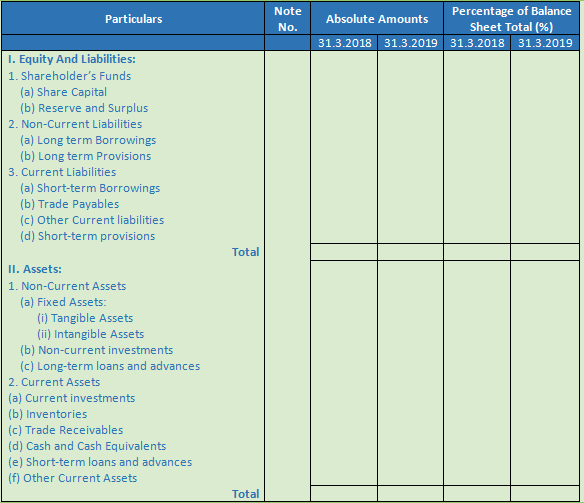Question .5.    Give a format of Common size Statement of Profit and Loss for two years.

Solution . 5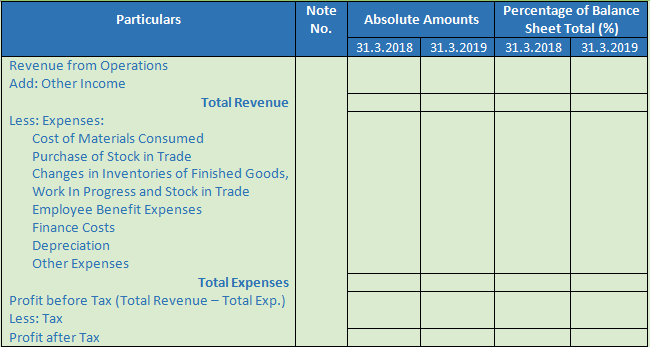Question .6.    Take a few imaginary figures and prepare a Common Size Statement of Profit and Loss for two years.

Solution . 6Question .7.    What is meant by Trend Percentage Analysis?

Solution . 7      Trend percentages are very useful in making comparative study of the financial statements for a number of years. These indicate the direction of movement over a long time and help an analyst of financial statements to form an opinion as to whether favourable or unfavourable tendencies have developed. This helps in future forecasts of various items.

Numerical Questions

Question .1.       Prepare Common size Balance Sheet of Vishal Paper Ltd. from the following information: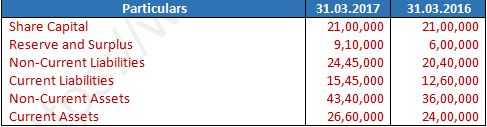Solution . 1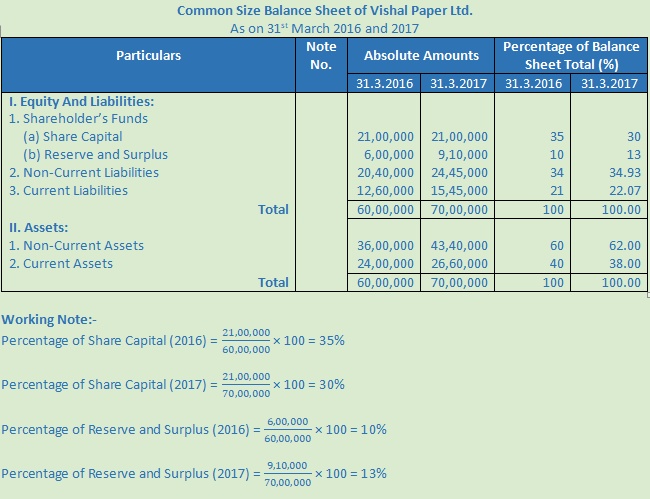Question .2.       The following balance sheets relate to Modern Computers Ltd. convert these into common size balance sheet and interpret the same: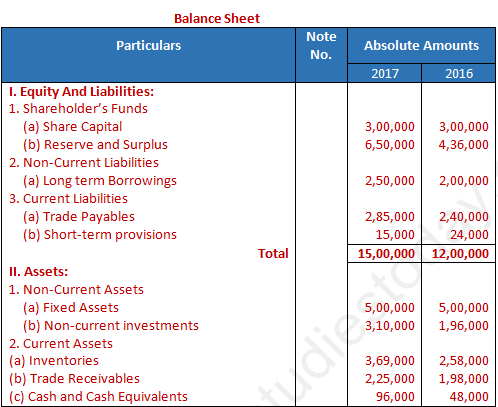Solution . 2Interpretations:

The analysis of the above comparative balance sheet gives the following conclusions:

(i) Current Assets for the year of 2016 were (21.50 + 16.50 + 4.00) 42% of the total assets and it is increased to (24.60 + 15 + 6.40) 46% in 2017.

(ii) Current liabilities for the year of 2016 were (20.00 + 2.00) 22% of the total liabilities and it is decreased to (19.00 + 1.00) 20% in 2017.

Question .3.       Prepare a common size balance sheet and comment on the financial position of A Ltd. and B Ltd. The Balance Sheet of A Ltd. and B Ltd. as at 31.3.2018 are given below: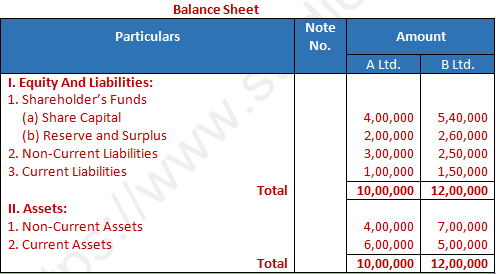Solution . 3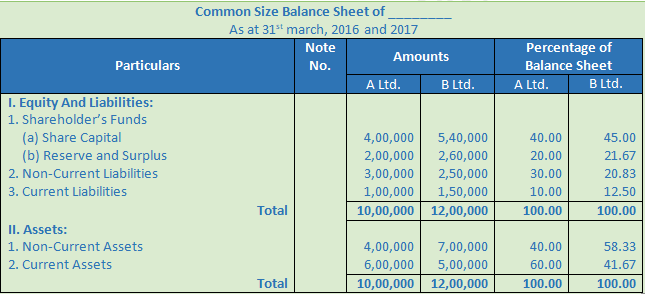Below are the observations in the financial position of A Ltd. and B Ltd. on the basis of above Common size balance sheet:

(i) The Share Capital of B Ltd is better as compared to A Ltd.

(ii) The position current liabilities of A Ltd. (10%) are better as compared to B Ltd. (12.50%)

(ii) The long term position of B Ltd. is better as compared to A Ltd.

(iv) The non-current assets of B Ltd. is 18.33% more as compared to A Ltd.

Question .4.     Prepare a Common Size Statement of Profit and Loss from the following Statement of Profit and Loss: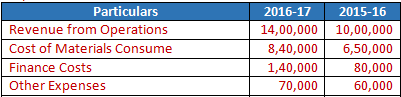Solution . 4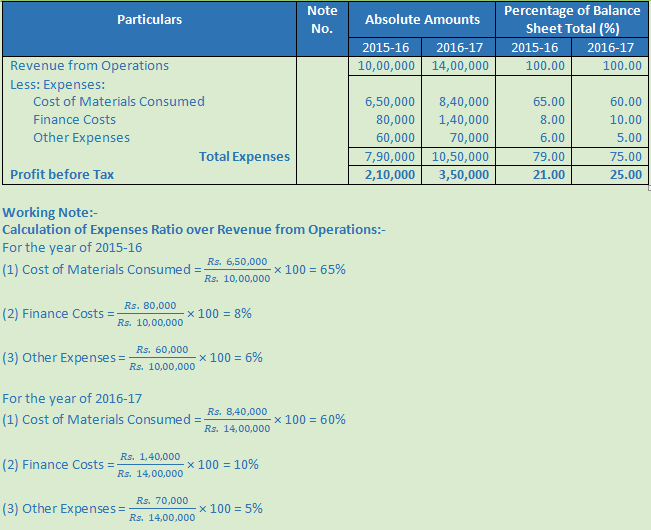Question .5.     From the following Statement of Profit and Loss prepare a Common Size Statement of Profit and Loss of Jayant Ltd. for the year ended 31.3.2018: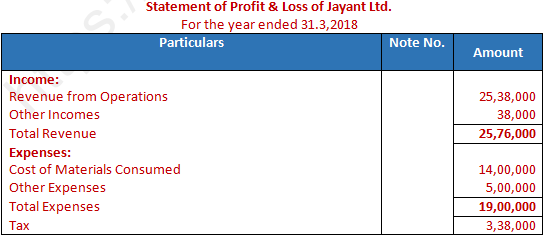Solution .5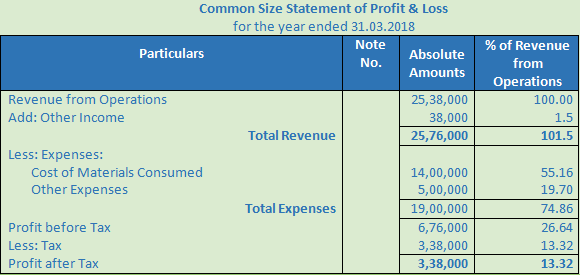Question .6.     Convert the following particulars into Common Size Statement of Profit and Loss and interpret the changes in 2018: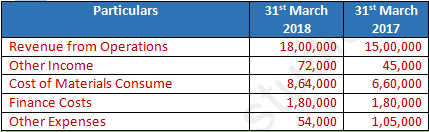Solution . 6Interpretation:-

The analysis of the above common Size Statement of Profit and loss gives the following conclusions:

(i) Cost of Material Consumed increased by 4% in 2018, which is 48% in 2018 and 44% in 2017. This increment due to cost of raw materials.

(ii) Employee Benefit Expenses reduce by 2% in 2018, which is 12% in 2018 and 10% in 2017.

(iii) Total Expenses reduce by 2% in 2018, which is 63% in 2018 and 61% in 2017. This result of companies better growth and efficiency.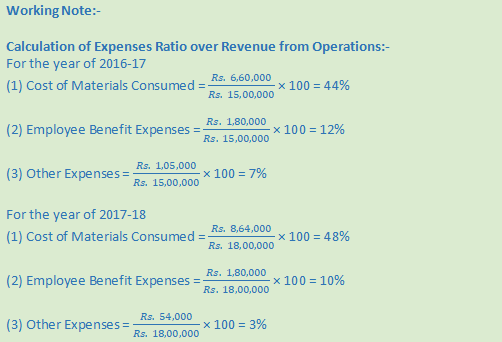Question .7.     Prepare a Common Size Statement of Profit and Loss from the following :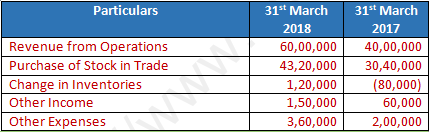Solution . 7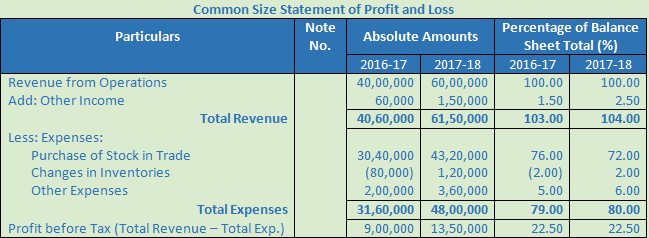Question .8.     Prepare a Common Size Statement of Profit and Loss from the following information of X Ltd.Solution . 8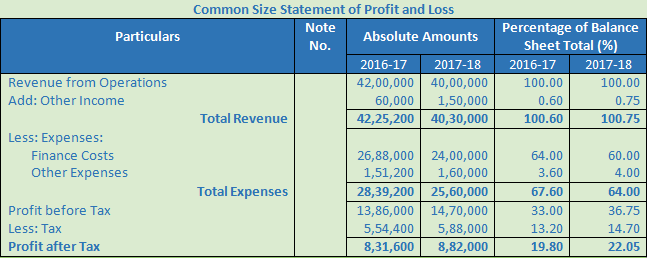Question .9.     Calculate the trend percentages from the following information taking year ending 2015 as the base year: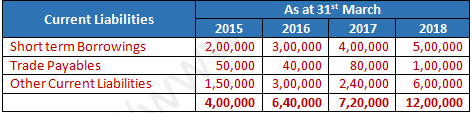Solution . 9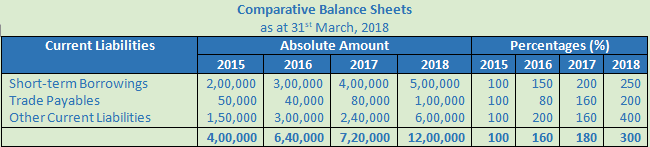Question .10.     From the following Balance Sheet of Sun Ltd. as at 31st March, 2017 prepare a common size Balance Sheet.Solution . 10Question .11.     Prepare a Common Size Balance Sheet of X Ltd. from the following information:Solution . 11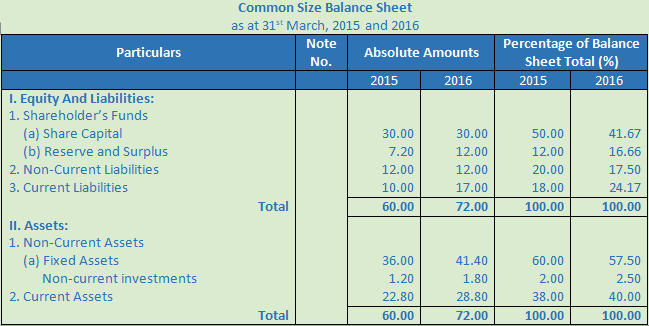Question .12.     From the following Balance Sheet of Vijay Ltd. as at 31st March, 2018 and 2017, prepare a common size Balance Sheet: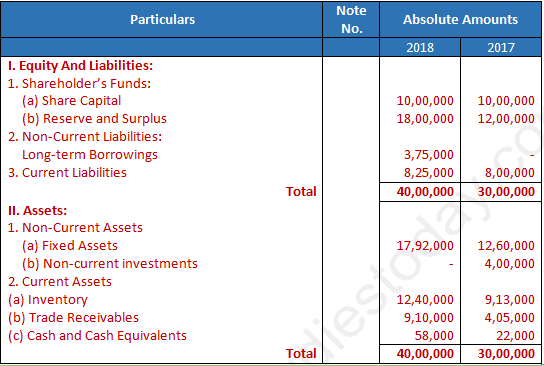Solution . 12Question .13.     From the following information, prepare a Common Size Balance Sheet and comment upon the changes: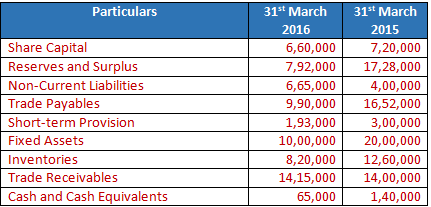Solution . 13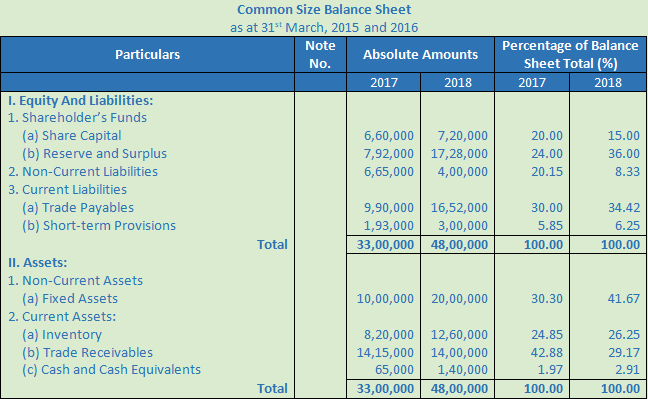Question .14.   Prepare a Common Size Balance Sheet and comment on the financial position of X Ltd. and Y Ltd. The Balance Sheet of X Ltd. and Y Ltd. as at 31st March, 2018 are given below: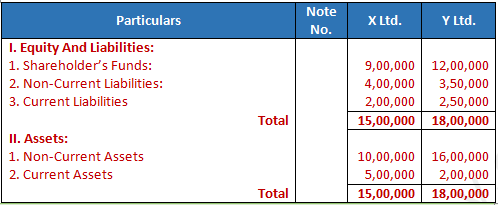Solution . 14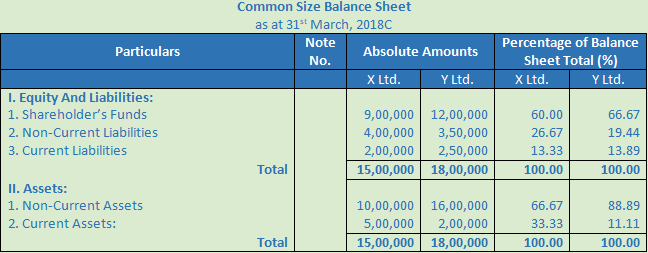Below are the observations in the financial position of X Ltd. and Y Ltd. on the basis of above Common size balance sheet:

(i) The Shareholders Fund of Y Ltd is more as compared to X Ltd.

(ii) The Non-current liabilities of X Ltd. (26.67%) are more B Ltd. (19.44%)

(ii) The Current Liabilities of X Ltd. and Y Ltd. are almost same.

(iv) The Non-current assets of Y Ltd. is 89.89% more as compared to X Ltd.

Question .15.     Prepare a Common Size Statement of Profit and Loss of X Ltd. from the following information:Solution . 15Question .16.     You are given the following information: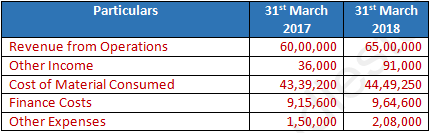Solution . 16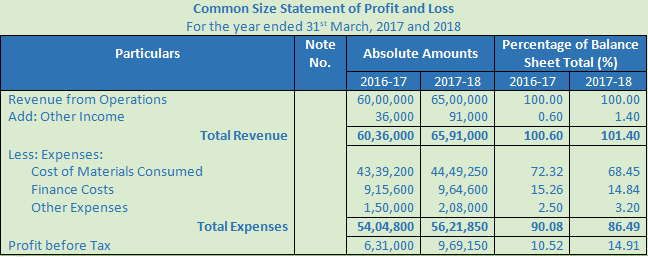Question .17.     Prepare a Common Size Statement of Profit and Loss from the following: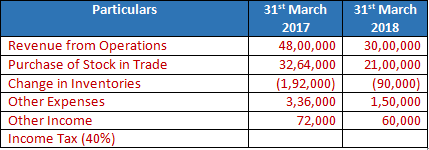Solution . 17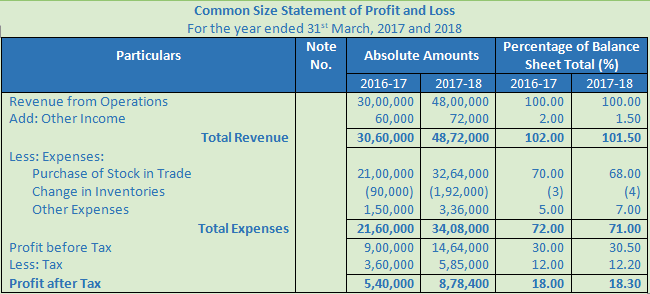Question .18.     Prepare a Common Size Statement of Profit and Loss of Vinod Paper Mills Ltd. from the following information: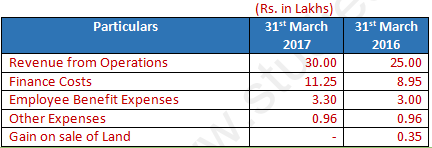Solution . 18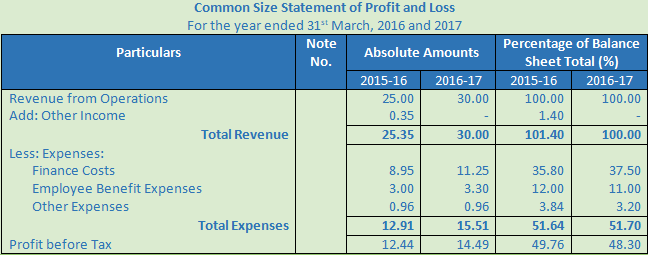Question .19.     Prepare a Common Size Statement of Profit and Loss from the following:Solution . 19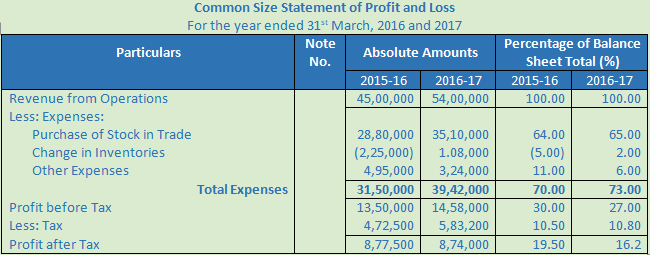Question .20.   From the following Statement of Profit and Loss of Star Ltd. for the year ended 31st March, 2018 prepare a Common Size Statement of Profit and Loss: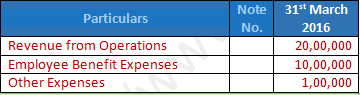Solution . 20Question .21.     From the information given below, prepare a Common Size Statement of Profit and Loss of Relay Ltd.:Solution . 21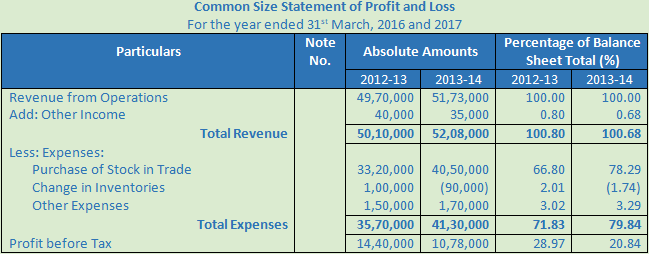Point of Knowledge:-

Trend percentages are very useful in making comparative study of the financial statements for a number of years. These indicate the direction of movement over a long time and help an analyst of financial statements to form an opinion as to whether favourable or unfavourable tendencies have developed. This helps in future forecasts of various items.